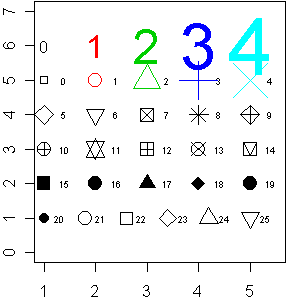# Producing Simple Graphs with R

The following is an introduction for producing simple graphs with the R Programming Language. Each example builds on the previous one. The areas in bold indicate new text that was added to the previous example. The graph produced by each example is shown on the right.

## Line Charts

First we'll produce a very simple graph using the values in the car vector:
```# Define the cars vector with 5 values
cars <- c(1, 3, 6, 4, 9)

# Graph the cars vector with all defaults
plot(cars)
```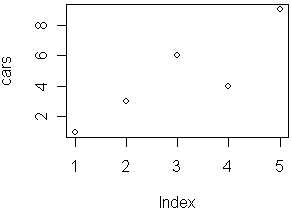Let's add a title, a line to connect the points, and some color:
```# Define the cars vector with 5 values
cars <- c(1, 3, 6, 4, 9)

# Graph cars using blue points overlayed by a line
plot(cars, type="o", col="blue")

# Create a title with a red, bold/italic font
title(main="Autos", col.main="red", font.main=4)
```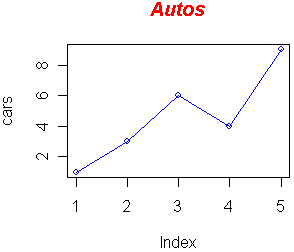Now let's add a red line for trucks and specify the y-axis range directly so it will be large enough to fit the truck data:
```# Define 2 vectors
cars <- c(1, 3, 6, 4, 9)
trucks <- c(2, 5, 4, 5, 12)

# Graph cars using a y axis that ranges from 0 to 12
plot(cars, type="o", col="blue", ylim=c(0,12))

# Graph trucks with red dashed line and square points
lines(trucks, type="o", pch=22, lty=2, col="red")

# Create a title with a red, bold/italic font
title(main="Autos", col.main="red", font.main=4)
```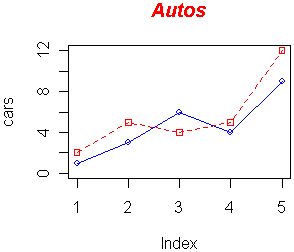Next let's change the axes labels to match our data and add a legend. We'll also compute the y-axis values using the max function so any changes to our data will be automatically reflected in our graph.
```# Define 2 vectors
cars <- c(1, 3, 6, 4, 9)
trucks <- c(2, 5, 4, 5, 12)

# Calculate range from 0 to max value of cars and trucks
g_range <- range(0, cars, trucks)

# Graph autos using y axis that ranges from 0 to max
# value in cars or trucks vector.  Turn off axes and
# annotations (axis labels) so we can specify them ourself
plot(cars, type="o", col="blue", ylim=g_range,
axes=FALSE, ann=FALSE)

# Make x axis using Mon-Fri labels
axis(1, at=1:5, lab=c("Mon","Tue","Wed","Thu","Fri"))

# Make y axis with horizontal labels that display ticks at
# every 4 marks. 4*0:g_range is equivalent to c(0,4,8,12).
axis(2, las=1, at=4*0:g_range)

# Create box around plot
box()

# Graph trucks with red dashed line and square points
lines(trucks, type="o", pch=22, lty=2, col="red")

# Create a title with a red, bold/italic font
title(main="Autos", col.main="red", font.main=4)

# Label the x and y axes with dark green text
title(xlab="Days", col.lab=rgb(0,0.5,0))
title(ylab="Total", col.lab=rgb(0,0.5,0))

# Create a legend at (1, g_range) that is slightly smaller
# (cex) and uses the same line colors and points used by
# the actual plots
legend(1, g_range, c("cars","trucks"), cex=0.8,
col=c("blue","red"), pch=21:22, lty=1:2);
```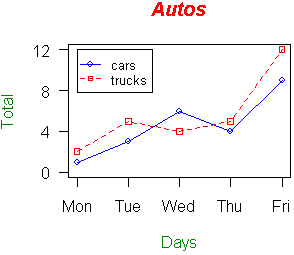Now let's read the graph data directly from a tab-delimited file. The file contains an additional set of values for SUVs. We'll save the file in the C:/R directory (you'll use a different path if not using Windows).

autos.dat

```cars	trucks	suvs
1	2	4
3	5	4
6	4	6
4	5	6
9	12	16
```
We'll also use a vector for storing the colors to be used in our graph so if we want to change the colors later on, there's only one place in the file that needs to be modified.  Finally we'll send the figure directly to a PNG file.
```# Read car and truck values from tab-delimited autos.dat

# Compute the largest y value used in the data (or we could
# just use range again)
max_y <- max(autos_data)

# Define colors to be used for cars, trucks, suvs
plot_colors <- c("blue","red","forestgreen")

# Start PNG device driver to save output to figure.png
png(filename="C:/R/figure.png", height=295, width=300,  bg="white")

# Graph autos using y axis that ranges from 0 to max_y.
# Turn off axes and annotations (axis labels) so we can
# specify them ourself
plot(autos_data\$cars, type="o", col=plot_colors,
ylim=c(0,max_y), axes=FALSE, ann=FALSE)

# Make x axis using Mon-Fri labels
axis(1, at=1:5, lab=c("Mon", "Tue", "Wed", "Thu", "Fri"))

# Make y axis with horizontal labels that display ticks at
# every 4 marks. 4*0:max_y is equivalent to c(0,4,8,12).
axis(2, las=1, at=4*0:max_y)

# Create box around plot
box()

# Graph trucks with red dashed line and square points
lines(autos_data\$trucks, type="o", pch=22, lty=2,
col=plot_colors)

# Graph suvs with green dotted line and diamond points
lines(autos_data\$suvs, type="o", pch=23, lty=3,
col=plot_colors)

# Create a title with a red, bold/italic font
title(main="Autos", col.main="red", font.main=4)

# Label the x and y axes with dark green text
title(xlab= "Days", col.lab=rgb(0,0.5,0))
title(ylab= "Total", col.lab=rgb(0,0.5,0))

# Create a legend at (1, max_y) that is slightly smaller
# (cex) and uses the same line colors and points used by
# the actual plots
legend(1, max_y, names(autos_data), cex=0.8, col=plot_colors,
pch=21:23, lty=1:3);

# Turn off device driver (to flush output to png)
dev.off()
```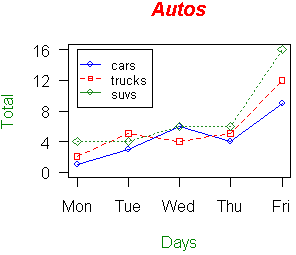In this next example, we'll save the file to a PDF and chop off extra white space around the graph; this is useful when wanting to use figures in LaTeX. We'll also increase the line widths, shrink the axis font size, and tilt the x-axis labels by 45 degrees.
```# Read car and truck values from tab-delimited autos.dat

# Define colors to be used for cars, trucks, suvs
plot_colors <- c(rgb(r=0.0,g=0.0,b=0.9), "red", "forestgreen")

# Start PDF device driver to save output to figure.pdf
pdf(file="C:/R/figure.pdf", height=3.5, width=5)

# Trim off excess margin space (bottom, left, top, right)
par(mar=c(4.2, 3.8, 0.2, 0.2))

# Graph autos using a y axis that uses the full range of value
# in autos_data. Label axes with smaller font and use larger
# line widths.
plot(autos_data\$cars, type="l", col=plot_colors,
ylim=range(autos_data), axes=F, ann=T, xlab="Days",
ylab="Total", cex.lab=0.8, lwd=2)

# Make x axis tick marks without labels
axis(1, lab=F)

# Plot x axis labels at default tick marks with labels at
# 45 degree angle
text(axTicks(1), par("usr") - 2, srt=45, adj=1,
labels=c("Mon", "Tue", "Wed", "Thu", "Fri"),
xpd=T, cex=0.8)

# Plot y axis with smaller horizontal labels
axis(2, las=1, cex.axis=0.8)

# Create box around plot
box()

# Graph trucks with thicker red dashed line
lines(autos_data\$trucks, type="l", lty=2, lwd=2,
col=plot_colors)

# Graph suvs with thicker green dotted line
lines(autos_data\$suvs, type="l", lty=3, lwd=2,
col=plot_colors)

# Create a legend in the top-left corner that is slightly
# smaller and has no border
legend("topleft", names(autos_data), cex=0.8, col=plot_colors,
lty=1:3, lwd=2, bty="n");

# Turn off device driver (to flush output to PDF)
dev.off()

# Restore default margins
par(mar=c(5, 4, 4, 2) + 0.1)
```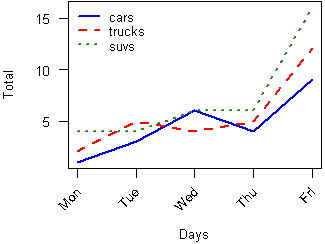## Bar Charts

```# Define the cars vector with 5 values
cars <- c(1, 3, 6, 4, 9)

# Graph cars
barplot(cars)
```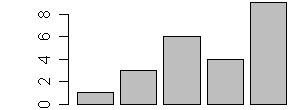Let's now read the auto data from the autos.dat data file, add labels, blue borders around the bars, and density lines:
```# Read values from tab-delimited autos.dat

# Graph cars with specified labels for axes.  Use blue
# borders and diagnal lines in bars.
barplot(autos_data\$cars, main="Cars", xlab="Days",
ylab="Total", names.arg=c("Mon","Tue","Wed","Thu","Fri"),
border="blue", density=c(10,20,30,40,50))
```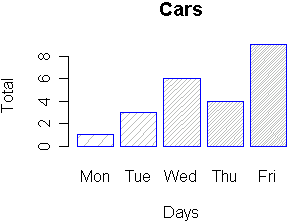Now let's graph the total number of autos per day using some color and show a legend:
```# Read values from tab-delimited autos.dat

# Graph autos with adjacent bars using rainbow colors
barplot(as.matrix(autos_data), main="Autos", ylab= "Total",
beside=TRUE, col=rainbow(5))

# Place the legend at the top-left corner with no frame
# using rainbow colors
legend("topleft", c("Mon","Tue","Wed","Thu","Fri"), cex=0.6,
bty="n", fill=rainbow(5));
```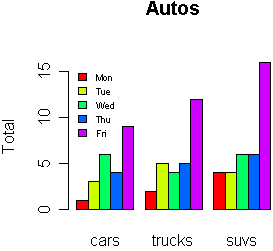Let's graph the total number of autos per day using a stacked bar chart and place the legend outside of the plot area:
```# Read values from tab-delimited autos.dat

# Expand right side of clipping rect to make room for the legend
par(xpd=T, mar=par()\$mar+c(0,0,0,4))

# Graph autos (transposing the matrix) using heat colors,
# put 10% of the space between each bar, and make labels
# smaller with horizontal y-axis labels
barplot(t(autos_data), main="Autos", ylab="Total",
col=heat.colors(3), space=0.1, cex.axis=0.8, las=1,
names.arg=c("Mon","Tue","Wed","Thu","Fri"), cex=0.8)

# Place the legend at (6,30) using heat colors
legend(6, 30, names(autos_data), cex=0.8, fill=heat.colors(3));

# Restore default clipping rect
par(mar=c(5, 4, 4, 2) + 0.1)
```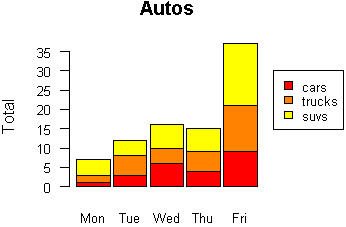## Histograms

Let's start with a simple histogram graphing the distribution of the suvs vector:
```# Define the suvs vector with 5 values
suvs <- c(4,4,6,6,16)

# Create a histogram for suvs
hist(suvs)
```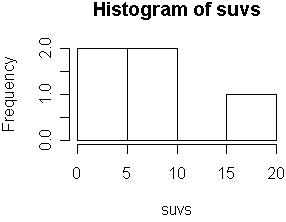Let's now read the auto data from the autos.dat data file and plot a histogram of the combined car, truck, and suv data in color.
```# Read values from tab-delimited autos.dat

# Concatenate the three vectors
autos <- c(autos_data\$cars, autos_data\$trucks,
autos_data\$suvs)

# Create a histogram for autos in light blue with the y axis
# ranging from 0-10
hist(autos, col="lightblue", ylim=c(0,10))
```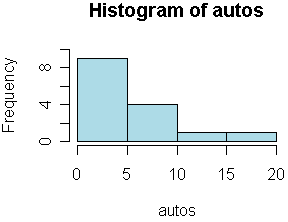Now change the breaks so none of the values are grouped together and flip the y-axis labels horizontally.
```# Read values from tab-delimited autos.dat

# Concatenate the three vectors
autos <- c(autos_data\$cars, autos_data\$trucks,
autos_data\$suvs)

# Compute the largest y value used in the autos
max_num <- max(autos)

# Create a histogram for autos with fire colors, set breaks
# so each number is in its own group, make x axis range from
# 0-max_num, disable right-closing of cell intervals, set
# heading, and make y-axis labels horizontal
hist(autos, col=heat.colors(max_num), breaks=max_num,
xlim=c(0,max_num), right=F, main="Autos Histogram", las=1)
```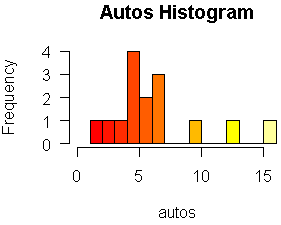Now let's create uneven breaks and graph the probability density.
```# Read values from tab-delimited autos.dat

# Concatenate the three vectors
autos <- c(autos_data\$cars, autos_data\$trucks,
autos_data\$suvs)

# Compute the largest y value used in the autos
max_num <- max(autos)

# Create uneven breaks
brk <- c(0,3,4,5,6,10,16)

# Create a histogram for autos with fire colors, set uneven
# breaks, make x axis range from 0-max_num, disable right-
# closing of cell intervals, set heading, make y-axis labels
# horizontal, make axis labels smaller, make areas of each
# column proportional to the count
hist(autos, col=heat.colors(length(brk)), breaks=brk,
xlim=c(0,max_num), right=F, main="Probability Density",
las=1, cex.axis=0.8, freq=F)

```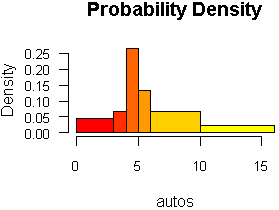In this example we'll plot the distribution of 1000 random values that have the log-normal distribution.
```# Get a random log-normal distribution
r <- rlnorm(1000)

hist(r)
```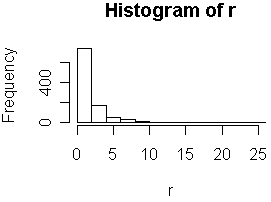Since log-normal distributions normally look better with log-log axes, let's use the plot function with points to show the distribution.
```# Get a random log-normal distribution
r <- rlnorm(1000)

# Get the distribution without plotting it using tighter breaks
h <- hist(r, plot=F, breaks=c(seq(0,max(r)+1, .1)))

# Plot the distribution using log scale on both axes, and use
# blue points
plot(h\$counts, log="xy", pch=20, col="blue",
main="Log-normal distribution",
xlab="Value", ylab="Frequency")

```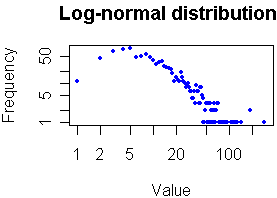## Pie Charts

```# Define cars vector with 5 values
cars <- c(1, 3, 6, 4, 9)

# Create a pie chart for cars
pie(cars)
```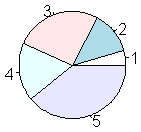Now let's add a heading, change the colors, and define our own labels:
```# Define cars vector with 5 values
cars <- c(1, 3, 6, 4, 9)

# Create a pie chart with defined heading and
# custom colors and labels
pie(cars, main="Cars", col=rainbow(length(cars)),
labels=c("Mon","Tue","Wed","Thu","Fri"))
```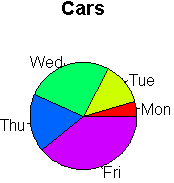Now let's change the colors, label using percentages, and create a legend:
```# Define cars vector with 5 values
cars <- c(1, 3, 6, 4, 9)

# Define some colors ideal for black & white print
colors <- c("white","grey70","grey90","grey50","black")

# Calculate the percentage for each day, rounded to one
# decimal place
car_labels <- round(cars/sum(cars) * 100, 1)

# Concatenate a '%' char after each value
car_labels <- paste(car_labels, "%", sep="")

# Create a pie chart with defined heading and custom colors
# and labels
pie(cars, main="Cars", col=colors, labels=car_labels,
cex=0.8)

# Create a legend at the right
legend(1.5, 0.5, c("Mon","Tue","Wed","Thu","Fri"), cex=0.8,
fill=colors)
```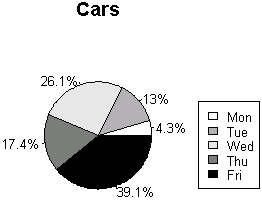## Dotcharts

```# Read values from tab-delimited autos.dat

# Create a dotchart for autos
dotchart(t(autos_data))
```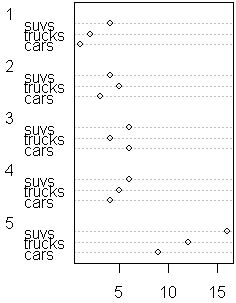Let's make the dotchart a little more colorful:
```# Read values from tab-delimited autos.dat

# Create a colored dotchart for autos with smaller labels
dotchart(t(autos_data), color=c("red","blue","darkgreen"),
main="Dotchart for Autos", cex=0.8)
```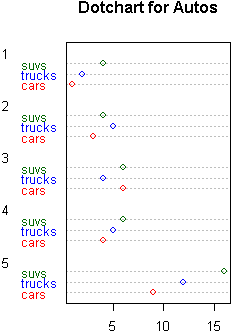## Misc

This example shows all 25 symbols that you can use to produce points in your graphs:
```# Make an empty chart
plot(1, 1, xlim=c(1,5.5), ylim=c(0,7), type="n", ann=FALSE)

# Plot digits 0-4 with increasing size and color
text(1:5, rep(6,5), labels=c(0:4), cex=1:5, col=1:5)

# Plot symbols 0-4 with increasing size and color
points(1:5, rep(5,5), cex=1:5, col=1:5, pch=0:4)
text((1:5)+0.4, rep(5,5), cex=0.6, (0:4))

# Plot symbols 5-9 with labels
points(1:5, rep(4,5), cex=2, pch=(5:9))
text((1:5)+0.4, rep(4,5), cex=0.6, (5:9))

# Plot symbols 10-14 with labels
points(1:5, rep(3,5), cex=2, pch=(10:14))
text((1:5)+0.4, rep(3,5), cex=0.6, (10:14))

# Plot symbols 15-19 with labels
points(1:5, rep(2,5), cex=2, pch=(15:19))
text((1:5)+0.4, rep(2,5), cex=0.6, (15:19))

# Plot symbols 20-25 with labels
points((1:6)*0.8+0.2, rep(1,6), cex=2, pch=(20:25))
text((1:6)*0.8+0.5, rep(1,6), cex=0.6, (20:25))
```